# Python 处理图像文件的实用姿势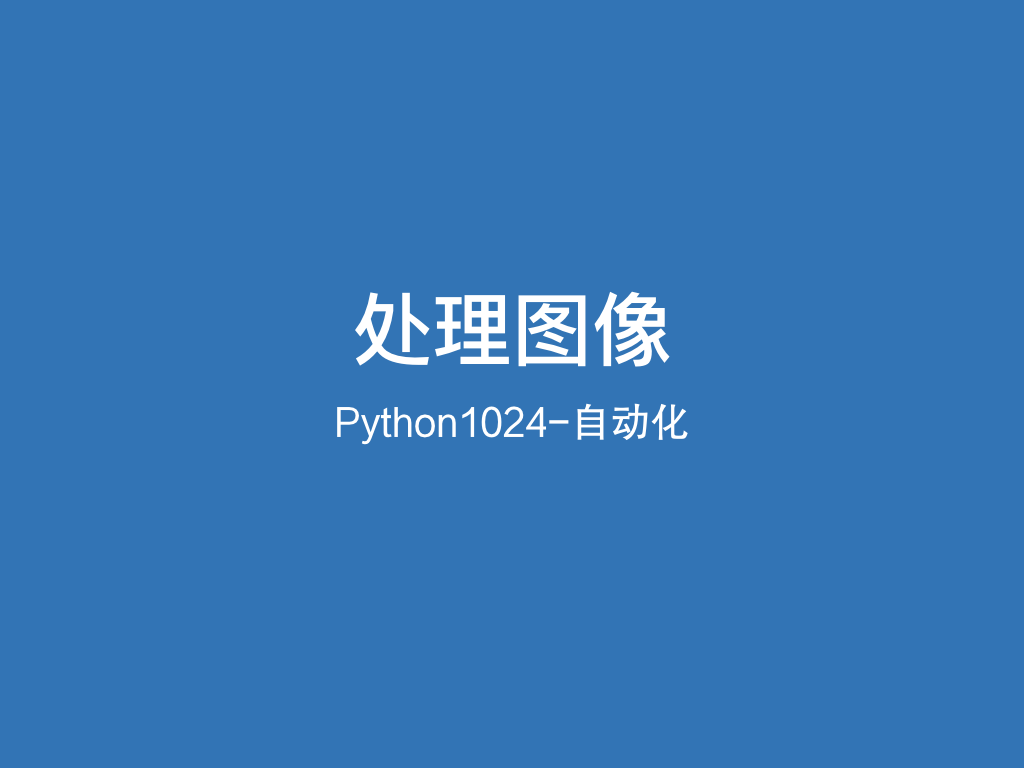# ﻿

﻿

“PS”技术不再局限于“电脑”，而且计算机慢慢开始拥有了自己的“视觉”能力。

1. 批量自动化处理图像，如裁剪、滤镜、旋转等效果叠加。

2. 智能识别图像内容，如人脸识别、人物识别等，用“PS”术语可以叫“自动抠图”。

﻿

Python处理图像的模块主要有3类：

• Pillow，即PIL最活跃分支，普及度高，提供多种图像格式处理能力。还有不少依赖于它的项目应用，如之前介绍的openpyxlpython-pptx，以及图像增强工具 Augmentor

• scikit-image，是科学计算生态scipy的图像能力补充。

• OpenCV，Intel发起的计算机视觉库，用C++实现后提供Python接口封装。

﻿

﻿

• 只做一些简单图片处理，比如大小、基本滤镜等，pillow足够。

• 需要灵活自定义高级滤镜，或研究图像算法，scikit-imageopencv更适合，有不少三方模块底层使用了opencv，所以根据具体应用情况可以穿插使用。只不过需要注意opencv内部数据不是RGB顺序，而是BGR顺序。

• 绘制图表，比如折线图、饼图等，不需要以上模块，用matplotlib更适合。

﻿

• pip install pillow

• pip install scikit-image

• pip install opencv

﻿

• 基本使用：打开、显示、保存，灰度化、锐化

• 数据互转：三个类库中图像数据互相转换

• 基本图像处理：调整大小、旋转、翻转、裁剪

• 图像滤镜：换底色、各类风格，如漫画、油画、漫画头像、复古、黑白

• 实战应用：屏幕截图、抠图、批量加水印

• 智能应用：人脸识别，如带口罩、情绪

## 基本使用

﻿

### Pillow基本使用

﻿

import pathlibfrom PIL import Image, ImageFilterpath = pathlib.Path('~/dev/python/python1024/data/automate/006image').expanduser()file_path = path.joinpath('lena.png')img = Image.open(file_path)print(img.size, img.mode, type(img))# img.show()img_gray = img.convert('L') # 灰度化img_gray.save(path.joinpath('006image_pillow_gray.png'))img_sharp = img.filter( ImageFilter.SHARPEN ) # 锐化img_sharp.save(path.joinpath('006image_pillow_sharp.png'))

﻿

###### scikit-image基本使用

﻿

import pathlibfrom skimage import io, colorfrom skimage.filters import unsharp_maskpath = pathlib.Path('~/dev/python/python1024/data/automate/006image').expanduser()file_path = path.joinpath('lena.png')img = io.imread(file_path)print(img.shape, type(img))# io.imshow(img) # 使用matplotlib显示图像img_gray = color.rgb2gray(img)io.imsave(path.joinpath('006image_skimage_gray.png'),img_gray)# enhanced image = original + amount * (original - blurred)img_sharp = unsharp_mask(img, radius=20, amount=1)io.imsave(path.joinpath('006image_skimage_sharp.png'),img_sharp)

﻿

### opencv基本使用

﻿

import pathlibimport cv2import numpy as nppath = pathlib.Path('~/dev/python/python1024/data/automate/006image').expanduser()file_path = path.joinpath('lena.png')img = cv2.imread(str(file_path))print(img.shape, type(img))# cv2.imshow('image',img)# cv2.waitKey(0)# cv2.destroyAllWindows()img_gray = cv2.cvtColor(img, cv2.COLOR_BGR2GRAY) # 灰化cv2.imwrite(str(path.joinpath('006image_cv2_gray.png')),img_gray)kernel = np.array([[-1,-1,-1],                   [-1, 9,-1],                   [-1,-1,-1]])img_sharp = cv2.filter2D(img, -1, kernel) # 锐化cv2.imwrite(str(path.joinpath('006image_cv2_sharp.png')),img_sharp)

﻿

## 三个模块间数据互转

﻿

﻿

import pathlibfrom PIL import Imageimport numpyimport cv2from skimage import io, img_as_float, img_as_ubytepath = pathlib.Path('~/dev/python/python1024/data/automate/006image').expanduser()file_path = path.joinpath('lena.png')img = Image.open(file_path)# PIL 转 opencvimg_cv = cv2.cvtColor(numpy.asarray(img),cv2.COLOR_RGB2BGR)print(img_cv.shape)# opencv 转 PILimg_back = Image.fromarray(cv2.cvtColor(img_cv,cv2.COLOR_BGR2RGB))# opencv 转 skimageimg_sk = img_as_float(cv2.cvtColor(img_cv,cv2.COLOR_BGR2RGB))print(img_sk.shape)# skimage 转 opencvimg_cv_back = cv2.cvtColor(img_as_ubyte(img_sk),cv2.COLOR_RGB2BGR)# skimage 转 PILimg_sk2pil = Image.fromarray(img_as_ubyte(img_sk))# PIL 转 skimageimg_pil2sk = img_as_float(img_sk2pil)print(img_sk.shape)

﻿

## 基本图像处理

﻿

Image是pillow最基础也是最核心的类，提供了读写文件、显示、格式转换、裁剪、合并、变形等一系列的功能。

﻿

import pathlibfrom PIL import Imagepath = pathlib.Path('~/dev/python/python1024/data/automate/006image').expanduser()file_path = path.joinpath('lena.png')img = Image.open(file_path)print(img.format, img.size, img.mode)# 创建一个缩略图，大小128x128size = (128, 128)img.thumbnail(size) # 不能放大，同时保持比例img.save(path.joinpath('006image_thumb.png'), 'PNG')img_resize = img.resize((512, 512)) # 自由缩放，放大后会模糊img_resize.save(path.joinpath('006image_resize.png'))img = Image.open(file_path) # 重新打开# 旋转img_rot = img.rotate(45)img_rot.save(path.joinpath('006image_rotate.png'))# 翻转img_all = Image.new('RGB', (512*3, 512*2), (0, 0, 0)) # 创建一个三行两列容器trans_list = [Image.FLIP_LEFT_RIGHT, Image.FLIP_TOP_BOTTOM,              Image.ROTATE_90,Image.ROTATE_180, Image.ROTATE_270]img_all.paste(img, (0,0))for i, trans in enumerate(trans_list, start=1):    x = (i%3)*512    y = (i//3)*512    img_trans = img.transpose(trans)    img_all.paste(img_trans, (x, y))img_all.save(path.joinpath('006image_transpose.png'))# 裁剪box = (100, 100, 400, 400) #（左、上、右、下）左上角坐标(0,0)img_crop = img.crop(box)img_crop.save(path.joinpath('006image_crop.png'))img_crop_mod = img_crop.transpose(Image.ROTATE_180)# 合并img.paste(img_crop_mod, box)img.save(path.joinpath('006image_merge.png'))

﻿

## 图像滤镜

﻿

• RGB常用于显示器，用红（Red）、绿（Green）、蓝（Blue）三色组合出色彩。

• CMYK常用于打印机，青（Cyan）、品红（Magenta）、黄（Yellow）和黑（blacK，避免与Blue混淆）。

• YCbCr常用于视频，Y为颜色亮度比例，Cb和Cr表示蓝色和红色浓度偏移量比例。

﻿

图像的色彩处理，其实就是对其颜色数据的处理。

﻿

﻿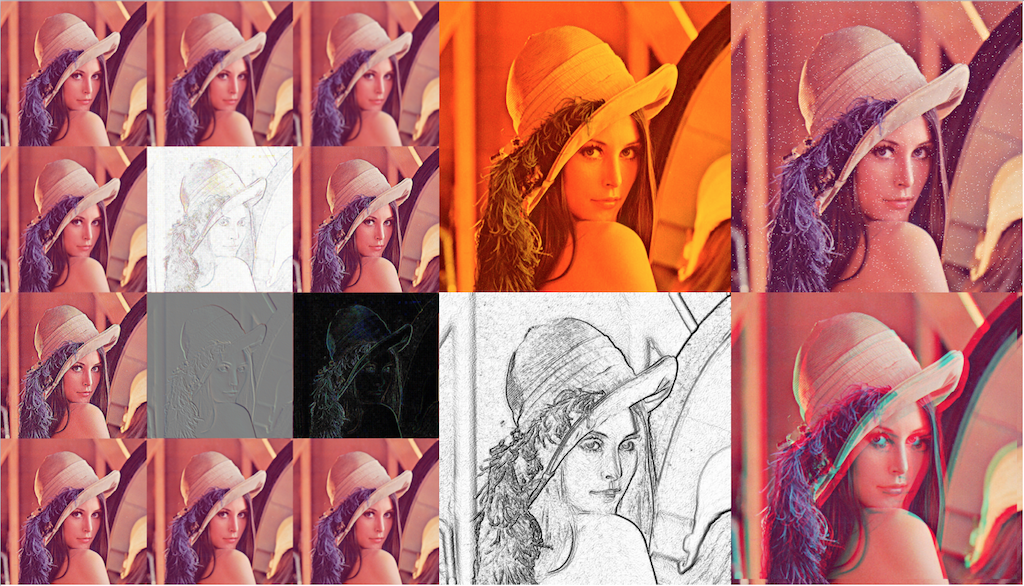﻿

﻿﻿

﻿

import pathlibfrom PIL import Image, ImageFilterimport numpy as nppath = pathlib.Path('~/dev/python/python1024/data/automate/006image').expanduser()file_path = path.joinpath('lena.png')img = Image.open(file_path)# mode就是采用的色彩模型print(img.format, img.size, img.mode)bands = img.getbands() # 返回所有通道bboxs = img.getbbox() # 返回左上角和右下角坐标extremas = img.getextrema() # 各个通道像素最小和最大值print(bands, bboxs, extremas)# 通道分离r, g, b = img.split()# 这里可以对每个通道的数据做些处理# 比如提高红色通道，降低绿色和蓝色通道，可以把图片变暖一些r = r.point(lambda x: x*1.3)g = g.point(lambda x: x*0.9)b = b.point(lambda x: 0)# 通道合并img_warm = Image.merge('RGB', (r, g, b))img_warm.save(path.joinpath('006image_warm.png'))# ImageFilter提供的预置滤镜filter_list = [ImageFilter.BLUR, ImageFilter.BoxBlur, ImageFilter.DETAIL,               ImageFilter.CONTOUR, ImageFilter.EDGE_ENHANCE, ImageFilter.EDGE_ENHANCE_MORE,               ImageFilter.EMBOSS, ImageFilter.FIND_EDGES, ImageFilter.SHARPEN,               ImageFilter.SMOOTH, ImageFilter.SMOOTH_MORE]img_all = Image.new('RGB', (512*3,512*4),(0,0,0))img_all.paste(img, (0,0))for i, filt in enumerate(filter_list, start=1):    if filt == ImageFilter.BoxBlur:        im = img.filter(filt(3))    else:        im = img.filter(filt)    x, y = 512*(i%3), 512*(i//3)    img_all.paste(im, (x,y))img_all.save(path.joinpath('006image_filters.png'))# 把图像转为numpy.array格式处理im = np.array(img)# 随机生成3000点盐白W, H = img.sizefor i in range(3000):    x=np.random.randint(0, W)    y=np.random.randint(0, H)    im[x,y,:]=255img_salt = Image.fromarray(im)img_salt.save(path.joinpath('006image_effect_salt.png'))# 底片效果im = np.array(img)im_gray = np.array(img.convert('L'))print(im.shape, im.dtype)print(im_gray.shape, im_gray.dtype)md_im = 255 - im_grayImage.fromarray(md_im).save(path.joinpath('006image_effect_photo.png'))# 手绘效果im_gray_float = im_gray.astype('float')# 深度值，范围为0～100# 深度值越大，图像梯度越大，体现为线条越多depth = 10grad_x, grad_y = np.gradient(im_gray_float) # 提取x和y方向的梯度值# 根据深度调整梯度值grad_x = grad_x*depth/100grad_y = grad_y*depth/100A = np.sqrt(grad_x**2 + grad_y**2 + 1.0)uni_x = grad_x/Auni_y = grad_y/Auni_z = 1.0/A# 引入光源vec_el = np.pi/2.2vec_az = np.pi/4.0dx = np.cos(vec_el)*np.cos(vec_az)dy = np.cos(vec_el)*np.sin(vec_az)dz = np.sin(vec_el)gd = 255*(dx*uni_x + dy*uni_y + dz*uni_z) # 光源归一化gd = gd.clip(0,255) #避免数据越界，将生成的灰度值裁剪至0-255之间im_out = Image.fromarray(gd.astype('uint8'))im_out.save(path.joinpath('006image_effect_pencial.png'))# 抖音效果im = np.array(img)im_r = np.copy(im)im_gb = np.copy(im)# 错位10个像素im_r[:-10, :-10, 1:3] = 0 # 去GB两个通道数据im_gb[10:, 10:, 0] = 0 # 去R通道数据im[:-10, :-10, :] = im_r[:-10, :-10, :] + im_gb[10:, 10:, :]img_out = Image.fromarray(im)img_out.save(path.joinpath('006image_effect_douyin.png'))# 如果连续生成不同错位像素的图片，就能拼成一个抖音效果的GIF动画img_dy_list = []for offset in range(2, 13, 2):    im = np.array(img)    im_r = np.copy(im)    im_gb = np.copy(im)    im_r[:-offset, :-offset, 1:3] = 0    im_gb[offset:, offset:, 0] = 0    im[:-offset, :-offset, :] = im_r[:-offset, :-offset, :] + im_gb[offset:, offset:, :]    img_dy_list.append(Image.fromarray(im))# Pillow 3.4以上（含）版本可以创建GIF图像img_dy_list.save(path.joinpath('006image_effect_douyin_gif.gif'), save_all=True,                    append_images=img_dy_list[1:], duration=100, loop=0)

﻿

## 实战应用

### 加水印和九宫格切图

﻿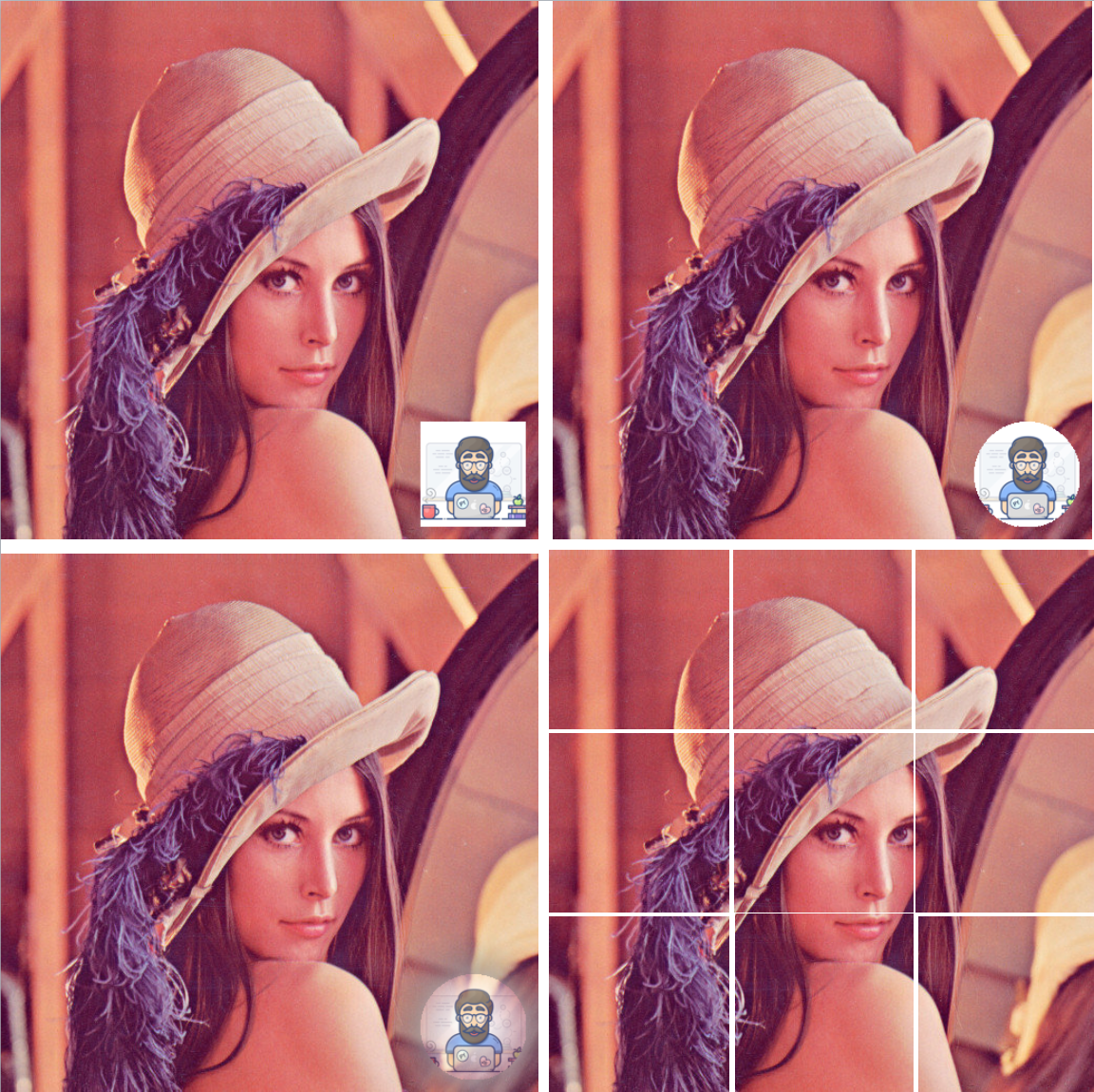﻿

pillow处理很方便：

﻿

import pathlibfrom PIL import Image, ImageDraw, ImageFontfrom PIL import ImageGrab, ImageFilterpath = pathlib.Path('~/dev/python/python1024/data/automate/006image').expanduser()file_path = path.joinpath('lena.png')img = Image.open(file_path)print(img.format, img.size, img.mode)# 屏幕截图img_screen = ImageGrab.grab((0,0,1024,300))# im = ImageGrab.grab() # 全屏img_screen.save(path.joinpath('006image_screen.png'))# 加文字水印font = ImageFont.truetype(str(path.joinpath('SourceHanSansCN-Bold.otf')), 20)img_txt = Image.new('RGBA', img.size, (0,0,0,0))draw = ImageDraw.Draw(img_txt)draw.text((300, 480), '程一初', font=font, fill=(255,255,255,255))img_txt = img_txt.rotate(45)# 两个图片必须具有相同的mode和sizeimg_wm = Image.alpha_composite(img.convert('RGBA'), img_txt)img_wm.save(path.joinpath('006image_txt_watermark.png'))# 加图片水印img_logo = Image.open(path.joinpath('avatar.jpg')).resize((100, 100))img_mask = Image.new('RGBA', img.size, (0,0,0,0))img_mask.paste(img_logo, (400,400))img_wm = Image.alpha_composite(img.convert('RGBA'), img_mask)img_wm.save(path.joinpath('006image_img_watermark.png'))# 把水印图片做成圆形，其实就是多加一层遮照logo_mask = Image.new('RGBA', img_logo.size, (0,0,0,0))draw = ImageDraw.Draw(logo_mask)draw.ellipse((0,0,100,100),fill=(0,0,0,255))img_logo_masked = Image.composite(img_logo, logo_mask, logo_mask)img_logo_masked.save(path.joinpath('006image_logo_circle.png'))img_mask = Image.new('RGBA', img.size, (0,0,0,0))img_mask.paste(img_logo_masked, (400,400))img_wm_circle = Image.alpha_composite(img.convert('RGBA'), img_mask)img_wm_circle.save(path.joinpath('006image_img_circle_watermark.png'))# 也可以加一些模糊处理img_mask_blur = img_mask.filter(ImageFilter.GaussianBlur(20))img_wm_circle_blur = Image.composite(img_mask, img.convert('RGBA'), img_mask_blur)img_wm_circle_blur.save(path.joinpath('006image_img_circle_blur_watermark.png'))# 九宫格切图，实际上应用的是Image.crop方法out_path = path.joinpath('006image_9cuts_out')if not out_path.is_dir():    out_path.mkdir()W, H = img.sizeW /= 3H /= 3for i in range(9):    x, y = (i%3)*W, (i//3)*H    img_out = img.crop((x,y,x+W,y+H))    img_out.save(out_path.joinpath(f'out_{i}.png'))

﻿

﻿

﻿

﻿

### 自动抠图

﻿

﻿

﻿

1. 计算出二值图像（即黑白图），每个像素点数据非0即1。

2. 根据二值图像为原图设置透明度，如把非人像部分全透明，再融合其他背景，就实现了换背景效果。

﻿

• 根据像素色彩范围，识别出背景。适合纯背景色场景，如证件照。

• 如果背景色彩复杂，就分块识别，在某小块中色彩相对集中。但需要单独设计切分算法。

• 机器学习算法，训练出识别物的特征模型。训练过程非常耗资源，但有了模型就可以快速应用，目前不少大平台也开放出了自己的训练模型，如百度的paddlehub项目。

﻿

﻿

# 一、把背景高于某个颜色的部分去掉import pathlibfrom PIL import Imagepath = pathlib.Path('~/dev/python/python1024/data/automate/006image').expanduser()file_path = path.joinpath('zjz.jpeg')img = Image.open(file_path).convert('RGBA')print(img.size, img.mode)W, H = img.size# 指定背景颜色，可以用多个点来增强精确度# 前提就是手工去获取背景的像素点颜色bg_color = (73, 112, 169, 255)bg_colors = [(64, 105), (80, 138), (116,190), 255]# 用python数组运行有点慢for w in range(W):    for h in range(H):        point = img.getpixel((w,h))        if point == bg_color: # 一个点效果太差            img.putpixel((w,h), (0,0,0,0))        elif all( bg_colors[i] < c < bg_colors[i]                for i, c in enumerate(point[:-1])):            img.putpixel((w,h), (0,0,0,0))img.save(path.joinpath('006image_pixel_alpha.png'))# 换个红底背景bg_red = Image.new('RGBA', img.size, 'red')bg_red = Image.alpha_composite(bg_red, img)bg_red.save(path.joinpath('006image_pixel_redbg.png'))

﻿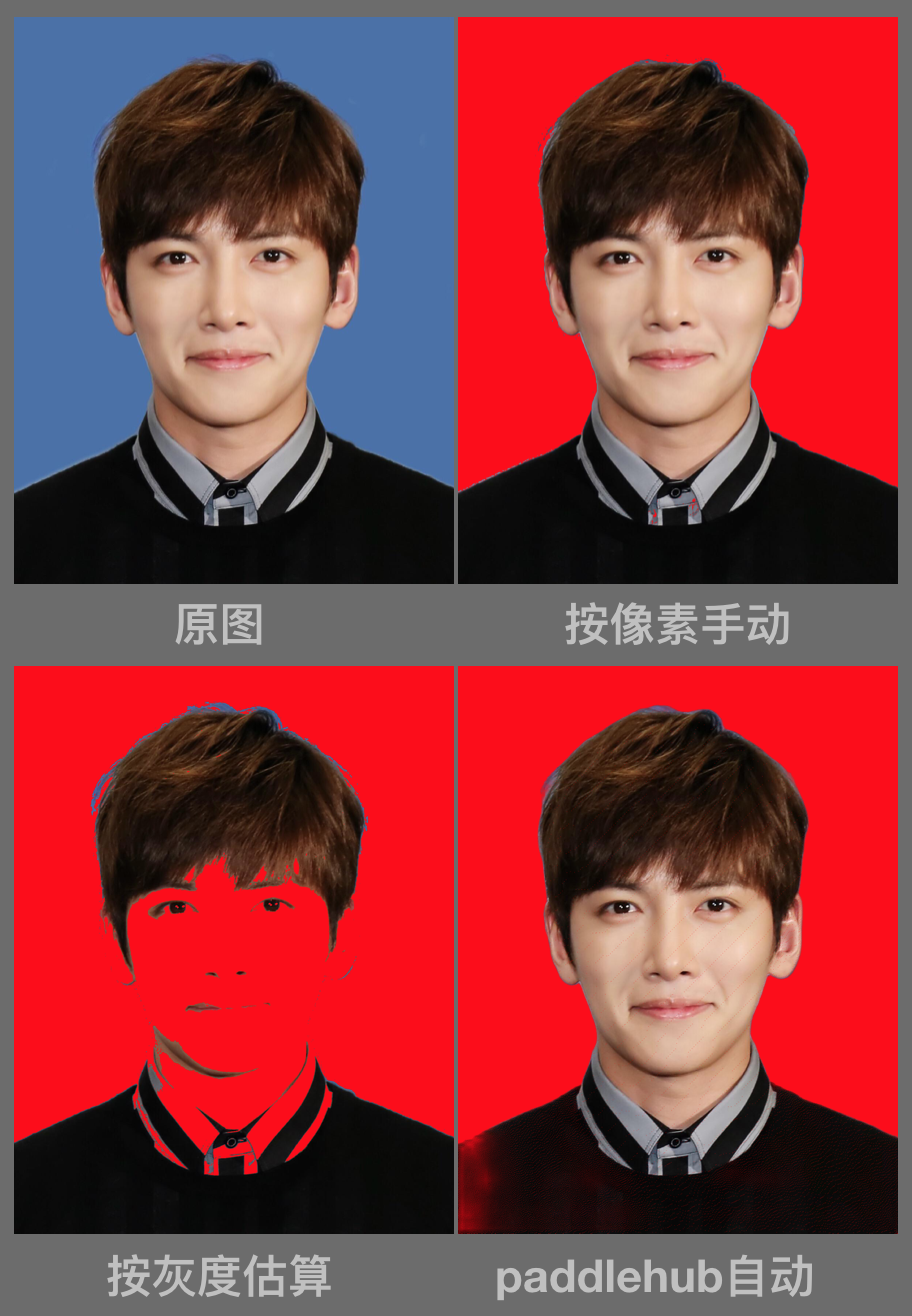﻿

• 按每个像素点色彩判断范围，需要手动指定背景色彩值范围，且用Python数组迭代效率低。

• opencvinRange函数生成二值数据，也要手动指定范围，但它支持位运算，效率高。

• 用灰度图，手动指定某个值来生成二值图，速度快，但精度差，效果当然也差。

• 使用paddlehub的人像模型识别，全自动，效果凑合，但资源损耗大，运算效率低。

﻿

## 智能应用

﻿

1. 引入模型：比如人像训练模型deeplabv3p_xception65_humanseg

2. 加载图片：指定文件路径

3. 算法执行：比如上面的图像切分segmentation

4. 输出结果

﻿

﻿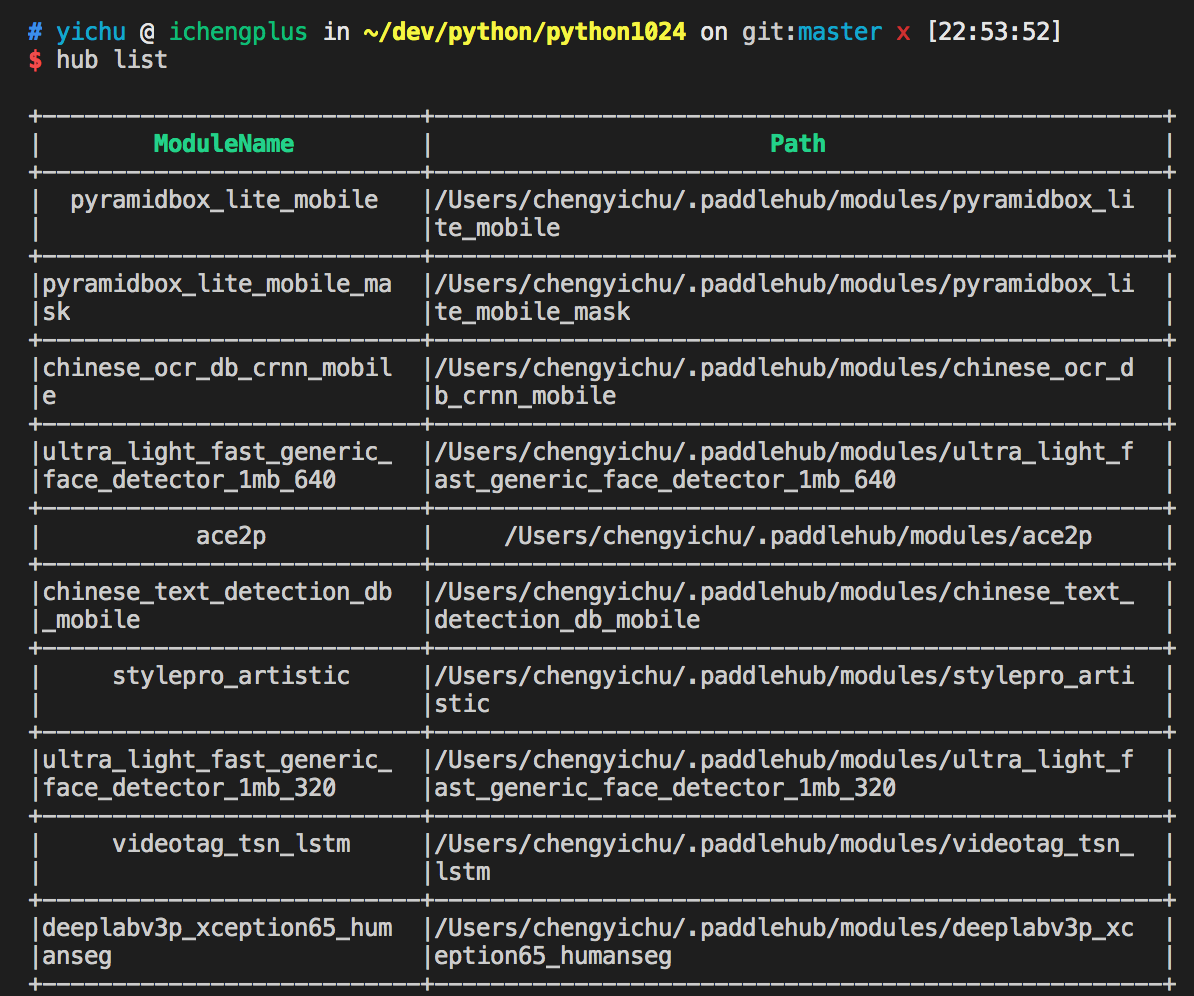﻿

import pathlibimport paddlehub as hubfrom PIL import Image, ImageDrawpath = pathlib.Path('~/dev/python/python1024/data/automate/006image').expanduser()file_list = [str(path.joinpath('mask1.jpg')), str(path.joinpath('mask2.jpg'))]out_path = path.joinpath('006image_face_out')module = hub.Module(name="pyramidbox_lite_mobile_mask")results = module.face_detection(data={"image": file_list})for res in results:    data = res['data']    img = Image.open(res['path'])    f_path = pathlib.Path(res['path'])    draw = ImageDraw.Draw(img)    for d in data:        draw.text((d['left'], d['top']-10), f'{d["label"]}: {d["confidence"]:.2%}', 'red')        draw.rectangle((d['left'], d['top'], d['right'], d['bottom']), outline='green', width=3)    img.save(out_path.joinpath(f_path.name))

﻿

﻿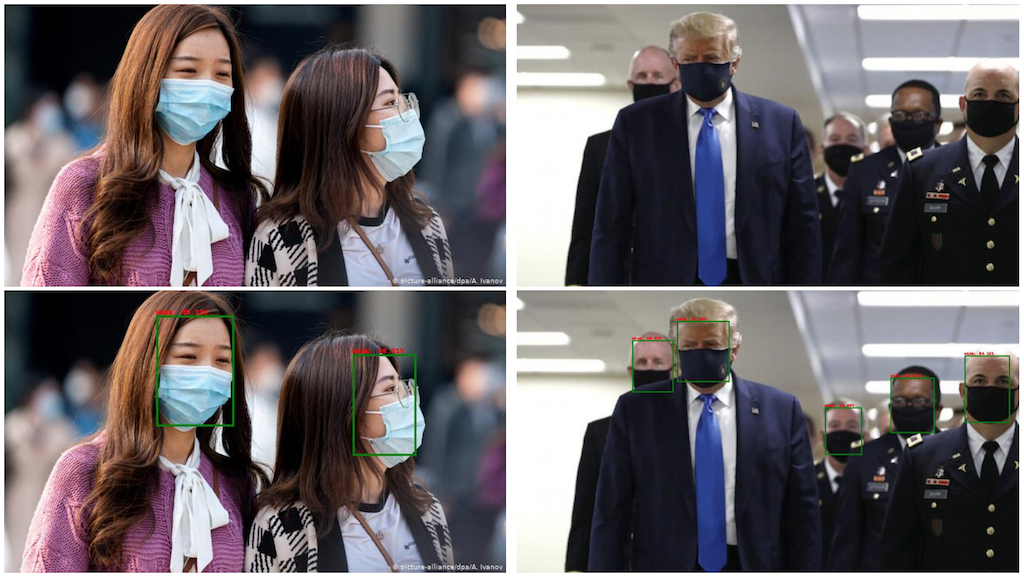﻿

## 总结

﻿

﻿

﻿

﻿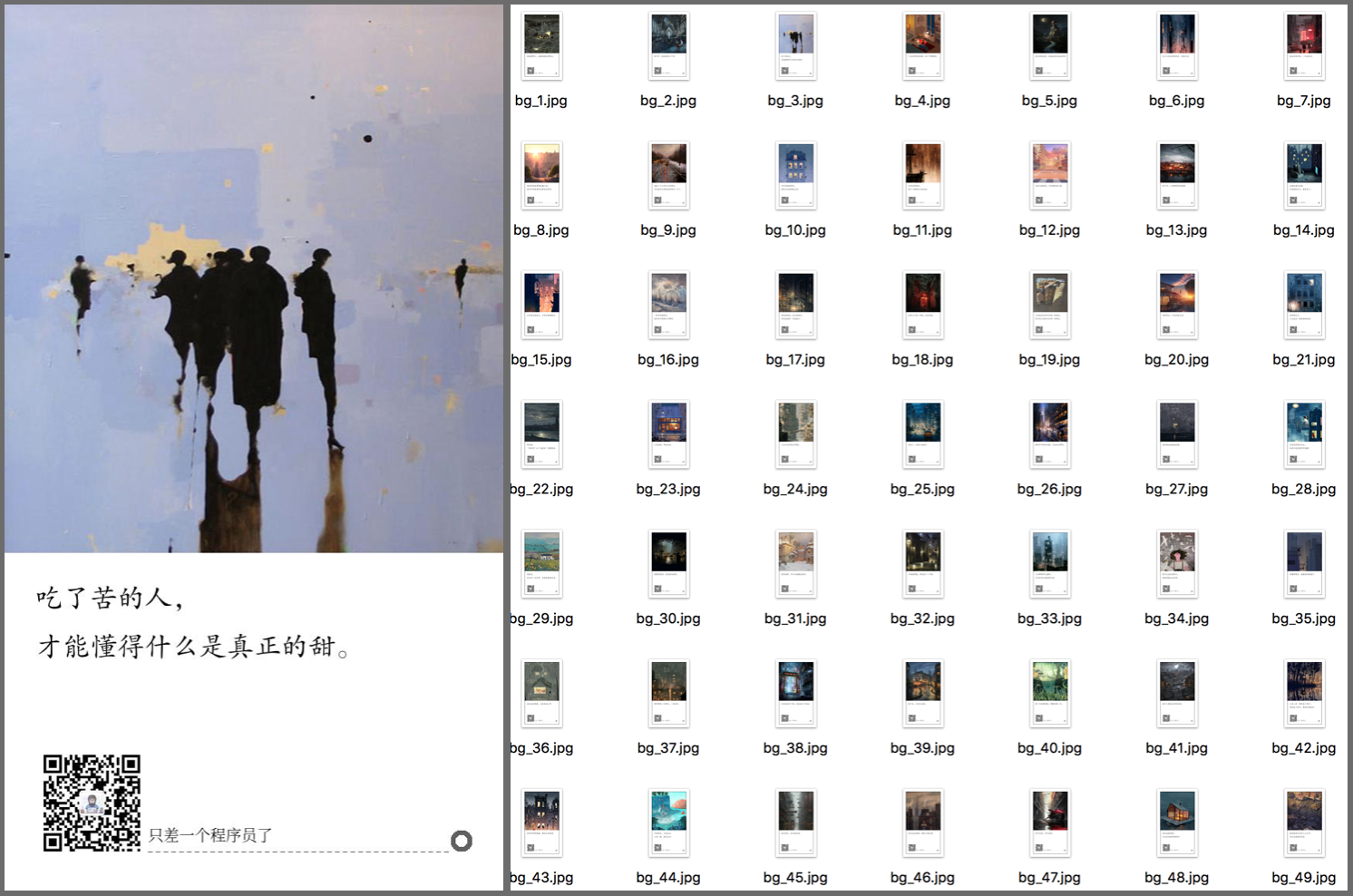﻿

﻿

﻿﻿## 评论